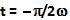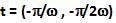## Thursday, June 10, 2010

### Irodov Problem 4.11Since the particle participates in two different simple harmonic motions its net motion is governed by their summation. The equation of motion of the particle is then given by,The velocity (1b) is thus the summation of two sine waves shown in the figure. As we trace time starting from t=0 towardswe see that both the sine waves are rising. Hence, from t=0 tothe velocity v will keep rising. After, one of the sine waves starts to fall while the other continues to rise. Thus, graphically it is clear that the maximum of velocity will lie somewhere at point A that lies in the intervalas shown in the figure.

Now the maximum of v will occur whenand. In other words,#### 1 comment:

1.